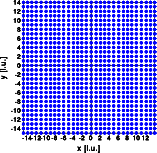Diffuse scattering: Longitudinal wavesDiffuse scattering
Thermal I
Thermal II
Occupational I
Occupational II
Longitudinal waves
Transversal waves
Short range order
Stacking faults

Interactive examples
Displacement waves
Short range order
Stacking faults

Goto
Contents

This example shows the effect of a longitudinal wave. The ideal crystal consists of a primitive cubic structure with one atom at 0,0,0. A longitudinal wave with wave vector displaces the atoms. The wave is characterized by:

 ```wave vector  wave length 27A 5.2 unit cells amplitude 0.5A 0.1 unit cells```

The left image shows a part of the crystal, 30*30 unit cells were used for the calculation. The right image shows one quadrant of reciprocal space.The satellite vector is 0.19 0 0, parallel to the wave vector in real space. Its absolute value is inversely proportional to the wave length. The intensity of the satellite reflections depends on the amplitude of the displacement. The satellites around the 0k0 reflections are systematically extinct. Here the term h ( u - < u > ) equals zero and no deviation from the averaged structure can be observed. Imagine the structure projected onto the 010 line. This projection is identical to that of a undisturbed crystal and accordingly the 0k0 reflections are identical.

© Th. Proffen and R.B. Neder, 2003